## 4. 1. 9 Eigenvalue Problems

The use of linearized expressions can also be generalized so as to be employed for the specification of eigenvalue equations. In this case, expressions of the form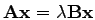the linearized expression can be specified. The Schrödinger equation which is typically used for the solution of quantum mechanical problems is a basic example for the treatment of eigenvalue equations using linear expressions.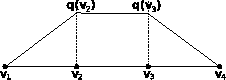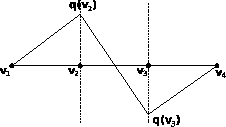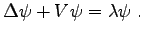(4.25)

The eigenvalue equation system can be written in the following line-wise form: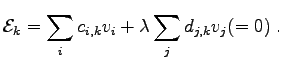(4.26)

For the sake of simplicity and in order to obtain a consistent short and concise notation comparable to linearized expressions (4.9), the following scheme is introduced: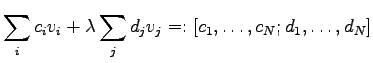(4.27)

As these eigenvalue equations are always linear, only linear operations have to be considered and a linear space is obtained.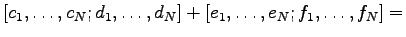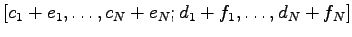(4.28)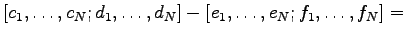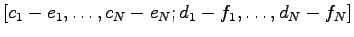(4.29)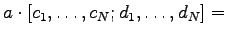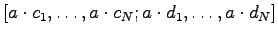(4.30)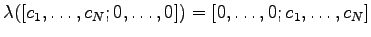(4.31)

An algebraic structure which introduces linear(ized) expressions to eigenvalue- expressions has to provide the following elements: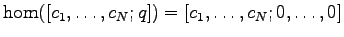(4.32)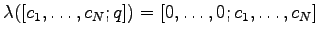(4.33)

The following discretization is obtained in a one-dimensional simulation domain according to Figure 4.1 comprising four vertices when using finite differences. The solution is referred to as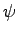which is stored in the quantity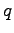. In analogy to (4.12), the term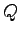is introduced.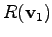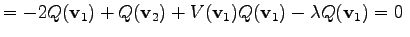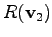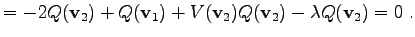(4.34)

The potential is assigned constant values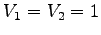. For the probability function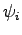the following term is inserted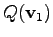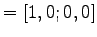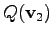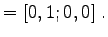(4.35)

Accordingly, one obtains the following eigenvalue expressions: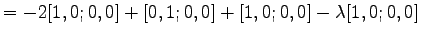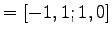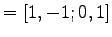(4.36)

In analogy to linearized expressions, linear eigenvalue expressions can be used in order to fill the eigenvalue matrices. One often observes that the matrix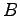is the unit matrix. Often it is necessary to change the order of the equations in order to maintain the unit matrix for.

Michael 2008-01-16